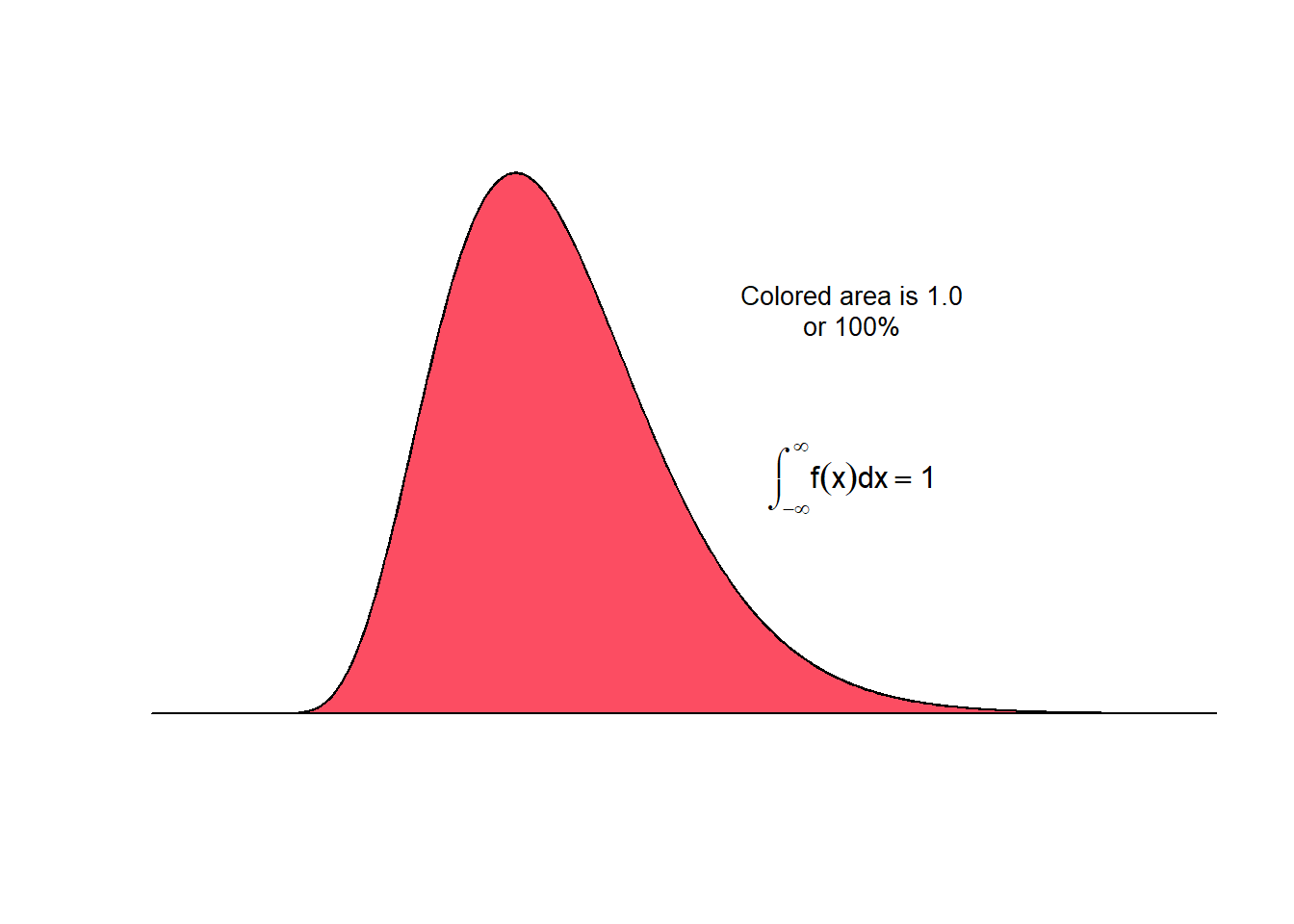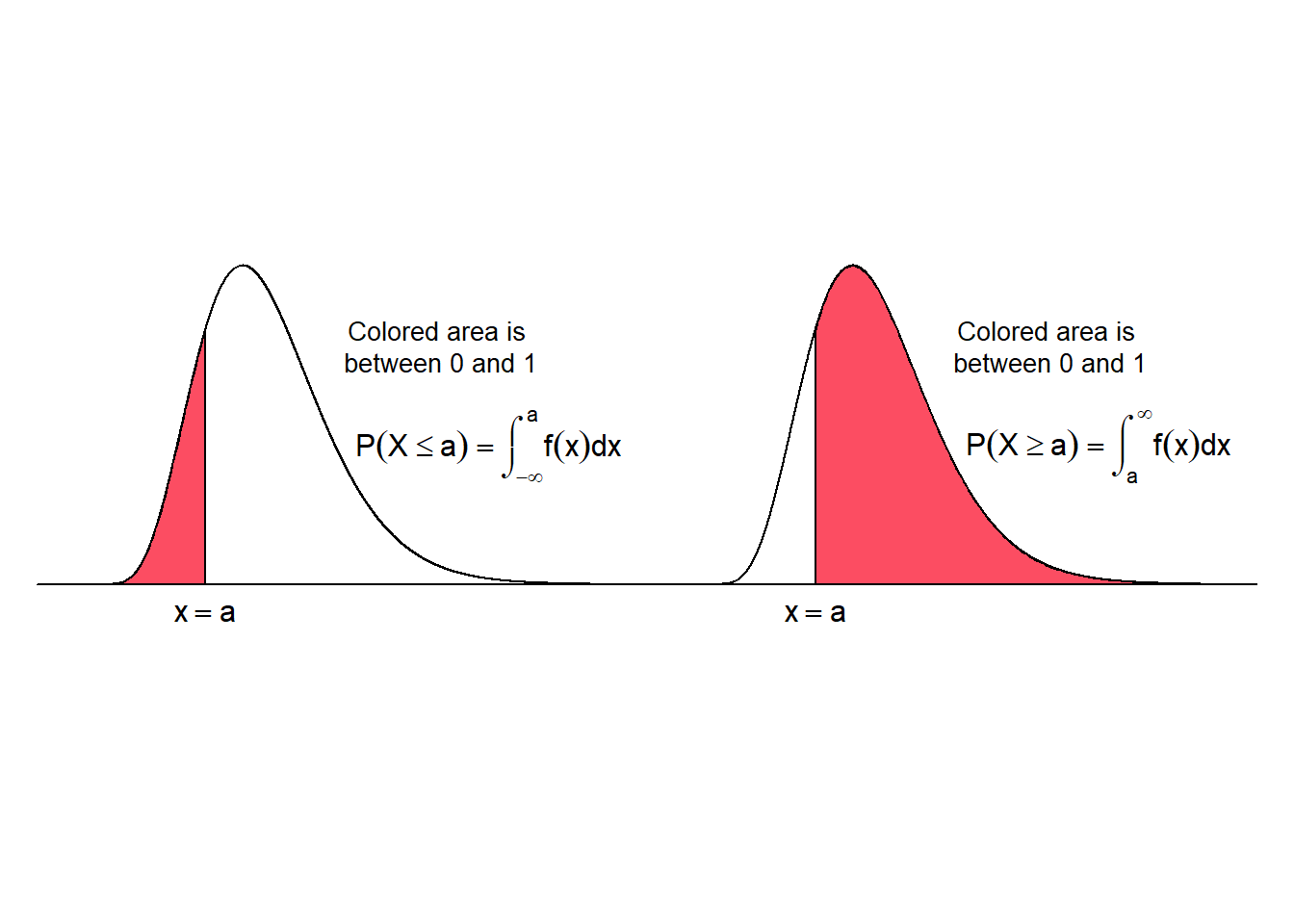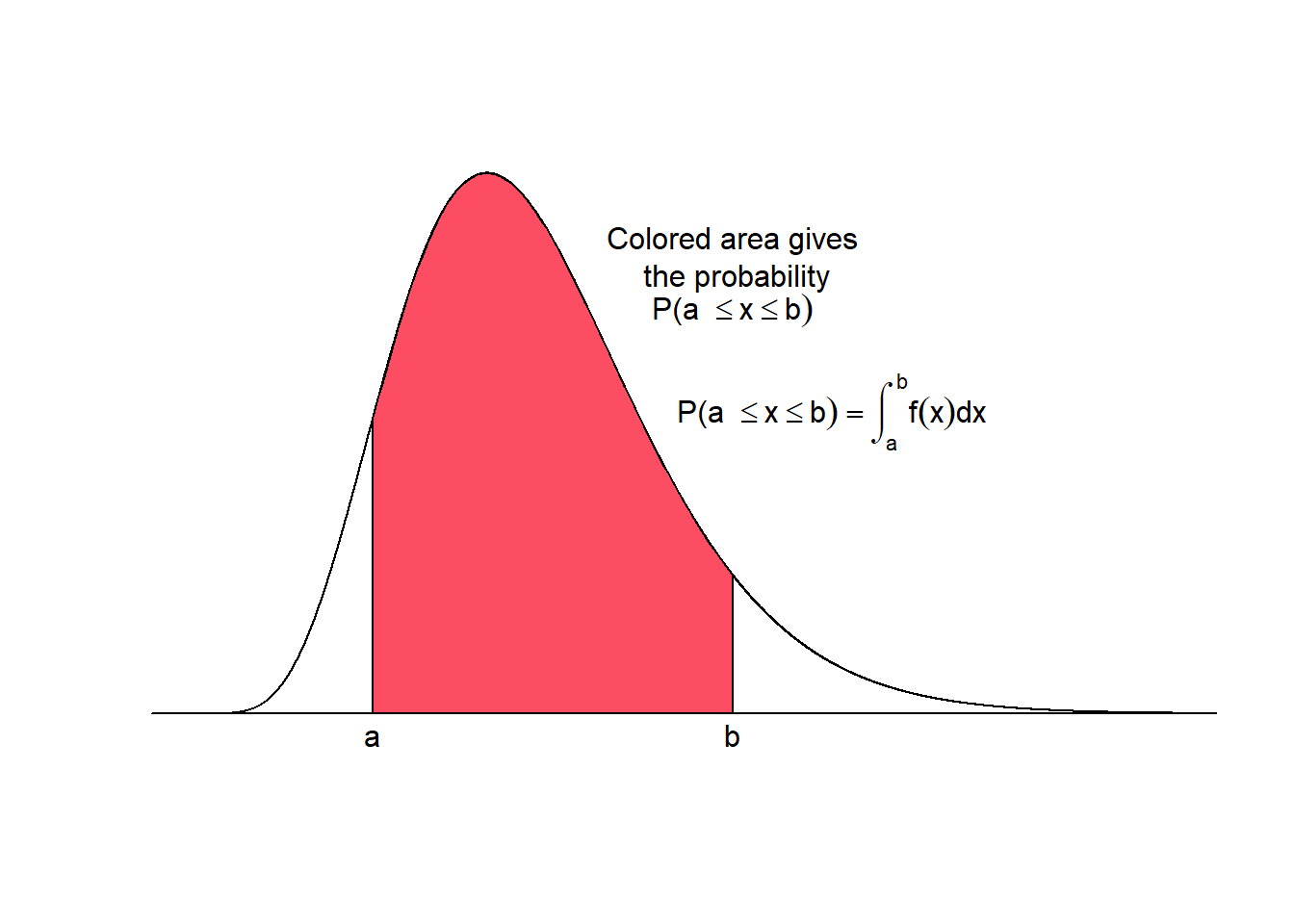The shape of the distribution of a random variable may be visualized with a smooth curve. Such curves, which represent the distribution of continuous variables, are called probability density functions (PDF) or just density functions. Probability density functions have three main properties (Mann 2012 , Weiss 2010):

1. a PDF always plots on or above the horizontal axis
2. the total area under a PDF (and above the horizontal axis) equals 1, and thus any value in any interval lies in the range 0 to 1
3. all possible observations of the variable that lie within any specified range equal the corresponding area under the density function and can be expressed as percentages or proportions.

The area under the curve is computed by the integral of the value $$x$$ from $$-\infty$$ to $$+\infty$$, which yields 1:

$\int_{-\infty}^{+\infty} f(x)dx = 1$The probability that a continuous random variable $$x$$ takes a value within a certain interval is given by the area under the curve between the two limits of the interval. The colored area under the curve for the interval $$(-\infty, a]$$ (left panel) and for the interval $$[a,\infty)$$ (right panel) is shown in the figure below.

The probability that $$x$$ falls in the interval $$(-\infty, a]$$ is

$P(X \le a) = \int_{-\infty}^{a}f(x)dx$

and the probability that $$x$$ falls in the interval $$[a, \infty)$$ is

$P(X \ge a) = 1 - P(X \le a) = \int_{a}^{\infty}f(x)dx$The probability that a continuous random variable $$x$$ assumes a value within a certain interval is given by the area under the curve between the two limits of the interval. The colored area under the curve from $$a$$ to $$b$$ in the figure below gives the probability that $$x$$ falls in the interval $$[a,b]$$.

## Warning in par(mfrow = c(1, 1), default.par): argument 2 does not name a
## graphical parameter\begin{align} P(a \le x \le b) & = \int_{a}^{b}f(x)dx\\ & = P(x \le b) - P(x \le a) \\ & = \int_{-\infty}^{b}f(x)dx - \int_{-\infty}^{a}f(x)dx \end{align}

Note: The interval $$a \le x \le b$$ states that $$x$$ is greater than or equal to $$a$$ but less than or equal to $$b$$.

For a continuous probability distribution, the probability is always calculated for an interval. The probability that a continuous random variable $$x$$ assumes a single value is always zero. This is because the probability to pick exactly one value out of an infinite number of values $$\in \mathbb R$$ is zero. In a geometric sense this means that the area of a line, which represents a single point, is zero.

$P(x) = 0$

From this we can deduce the following for a continuous random variable:

$P(a \le x \le b) = P(a < x < b)$

In other words, the probability that $$x$$ assumes a value in the interval $$a$$ to $$b$$ is the same, whether or not the values $$a$$ and $$b$$ are included in the interval.

Citation

The E-Learning project SOGA-R was developed at the Department of Earth Sciences by Kai Hartmann, Joachim Krois and Annette Rudolph. You can reach us via mail by soga[at]zedat.fu-berlin.de.You may use this project freely under the Creative Commons Attribution-ShareAlike 4.0 International License.

Please cite as follow: Hartmann, K., Krois, J., Rudolph, A. (2023): Statistics and Geodata Analysis using R (SOGA-R). Department of Earth Sciences, Freie Universitaet Berlin.